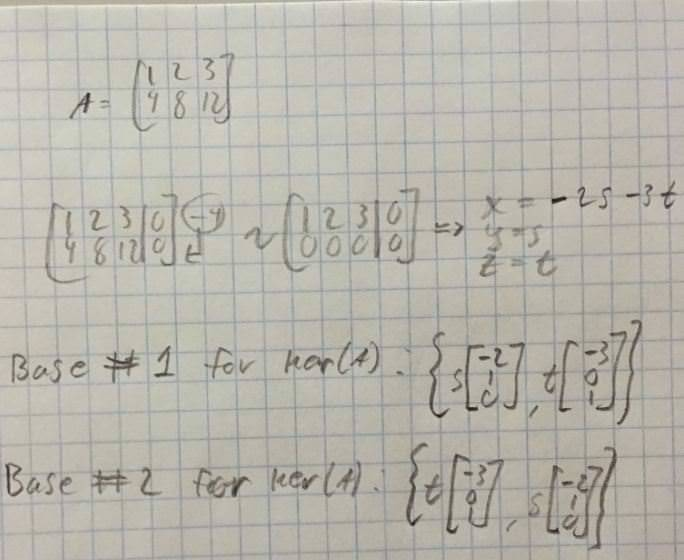# Determine a basis for kernel/nullspace

## Homework Statement

[/B]
A=##\begin{bmatrix}
1 & 2 & 3 \\
4 & 8 & 12
\end{bmatrix}##

Question: Determine a basis for ker(A).

## Homework Equations

Ker(A)=##\begin{bmatrix}
1 & 2 & 3& |&0 \\
4 & 8 & 12& |&0
\end{bmatrix}##

(And reduce with gaussian elimination)

## The Attempt at a Solution

(Sorry for no attempt on LaTeX here, it's my first time trying to use it at all above.)

Some background information, i am revisiting linear algebra and I were doing gram-schmidt process and got a totally different answer than the textbook, however i am confident i did not make a mistake during the actual gram-schmidt.
Then it occurred to me that i must have chosen a different basis than the textbook, which made me unsure in what i recall and know about the basis for the kernel. I couldn't really find any straight forward information about it in my textbook or with a few google searches, so now i've gotten caught up in my own minor insecurities in basic details.

A easy example below to illustrate my point, are both Basis #1 and Basis #2 correct (i just realised that i wrote base on the paper...)? They would of course lead to two very different orthogonal basis when using gram-schmidt, but both would be orthogonal nonetheless.

My reasoning behind them being equally correct is that there is no (to me) intuitive "basis vector 1 and basis vector 2" as it would be for example unit vectors. Still, the span should be independent of the order in the "set" anyway if i am not wrong? I.e. i would still reach all the points regardless of the order.#### Attachments

andrewkirk
Homework Helper
Gold Member
A basis is an unordered set of vectors. So the two things you wrote relate to the same basis, which is indeed a basis for the null space.

Also, you don't write the s and t in writing the basis. Writing the s and t gives an arbitrary element of the null space, not a basis. In this case a basis is just two purely numeric vectors.

•Bobman
A basis is an unordered set of vectors. So the two things you wrote relate to the same basis, which is indeed a basis for the null space.

Also, you don't write the s and t in writing the basis. Writing the s and t gives an arbitrary element of the null space, not a basis. In this case a basis is just two purely numeric vectors.
Great, thanks.

That's very true, i think i tried to make it overly clear with the "order" by keeping the parameters.

Thanks for clarifying!

Mark44
Mentor
Great, thanks.

That's very true, i think i tried to make it overly clear with the "order" by keeping the parameters.

Thanks for clarifying!
The basis vectors are the things that completely define this subspace. Every vector in the nullspace is a linear combination of these two vectors, so if ##\vec v \in Null(A)##, then ##\vec v = s<-2, 1, 0> + t<-3, 0, 1>##.
That's where the parameters come in, not when you are just listing the vectors that form a basis. Note that any nonzero multiple of either vector would also consitute a basis.
In a set, the order of the set elements doesn't matter.